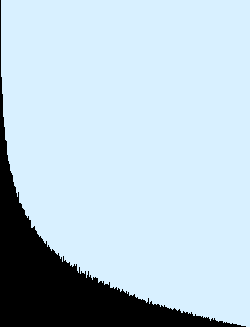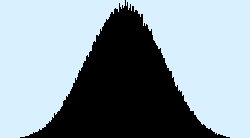Posted by: pixelero | April 24, 2008

## various functions with different distributions for Math.random()

All my earlier posts have mainly concerned flash and actionscript, this time something more general:

As a starting point we have Math.random() returning a value between 0.0 … 1,0 with even distribution:In case we want a random number more likely to be closer to like for instance 0.0, my old trick was to multiply Math.random()*Math.random, much greater odds to be <0.5.Maths with random are sometimes very unpredictable, in the case of Math.sqrt(Math.random)) we get an – almost ? – linear curve. Function random() has a 75% change of being >0.25, so squarerooted that’s 75% for >0.5, I think:very similar to Math.max(Math.random(), Math.random() ), greater of two random samples, with also 75% change for >0.5:Max of several randoms seems to approach a polynomial curve, just compare Math.max(Math.random(), Math.random(), Math.random()) with f(x)=x²:Using min instead of max naturally mirrors the situation towards 0.0.

As I mentioned this to be a bit unpredictable, when it comes to distribution random()+random() definately doesn’t equal 2*random(). In case we need randoms mainly on the mid-values, we use an average of two, 0.5*(Math.random()+Math.random()):nice pyramid that is !

Surprise, surprise, or maybe not if you’re good at statistics and probability maths: Taking an average of even more numbers approaches the Gaussian distribution:
0.333*(Math.random()+Math.random()+Math.random()):0.25*(Math.random()+Math.random()+Math.random()+Math.random()):Addition and substraction gives similar results, except that naturally random()-random() is in the range between -1.0 … 1.0.
And finally a modified gaussian emphasizing the border values:

r=Math.random()-Math.random()+Math.random()-Math.random();
r += (r<0.0) ? 4.0 : 0.0;
r *= 0.25;And the inverse 0.2/Math.random() … , well test it yourself:

… small javascript-demo for testing

Just like in the example function here, my demo shows only the values in the range 0.0…1.0, so add a proper scaling factor and addition in case you can’t see anything ! … and, hey, please comment if you come up with something interesting !

The pics in this posting were done with a code taking 20 times more samples, and a whole lot slower, than the linked demo, so don’t wonder if your result looks more rough !

## Responses

1.one more:

f= 0.2; r=f*Math.random()+(1.0-f)*Math.random()*Math.random(); // peak in x=f, value f<0.5

2.That’s some funny coincidence – I’ve just made some very similar experiments with the BitmapData.noise() method. You didn’t by chance read the “Good Math, Bad Math” blog article about random distributions?

3.“Maths with random are sometimes very unpredictable”

That is totally the point! 🙂

4.Mario: Experimenting this with BitmapData.noise() – or perlinNoise()? – sounds interesting. I did already wonder how to extend and utilize this in 2D, 3D …

With google I found some older writings of ‘gaussian’ math.random, but I didn’t see ‘Good math, bad math’ earlier – thanks for mentioning.

Jake: glad I made it clear — or just confused even more ! 🙂

5.I was playing with noise(), but I’m pretty sure that perlinNoise() might give some interesting results, too (except that perlinNoise doesn’t give you the full range of numbers from 0 – 255).

Looking at the histogram of BitmapData.noise() with low random seeds also reveals that that the random distribution for those seeds is not as “good” as for higher seeds.

6. […] various functions with different distributions for Math.random() « Pixeleroで興味深いことをやっていたのでFlashで実践。Math.random()は0以上1未満の乱数をだいたい均等な頻度で返す。じゃあ例えばこれは？ […]

7.Interesting…I just learned this in probability and statistics. It’s the central limit theorem right? The more random samples you take, the more likely you’ll have a Gaussian(bell-shaped) distribution.

8.@pixelero: Nice work.
@CJ CAT: Well, it´s while ago since my last econometric-lessons but I think this is the point;)

9.Pixelero, thanks a lot for publishing these functions. Very inspiring. They helped me a lot with finding more interesting ways of semi-randomly distributing forms on the digital canvas. Check out my latest experiments @ http://www.flickr.com/photos/dear_computer/

10. […] various functions with different distributions for Math.random() […]

11. […] – this is also an article by PIXELERO, the article is available here: various functions with different distributions for Math.random(). I’ve also added a greyscale representation underneath to the distributions as a series of […]

12.Grate one!) Loved idea, ported rendering to HTML5 canvas and modified gui a bit… hit enter on input feild to redraw=) http://jsdo.it/oleg.jakushkin/nZo3

•Thanks, very nice idea of updating it to html5/canvas !

13. […] one link leading most visitors to this blog is a discussion on a programming forum and that this old post of mine about random numbers is among the most popular ones. No wonder, there’s not much information about how to modify […]

14.function rndHill(skew){
let r = Math.sqrt(Math.random());
for (let i = 1; i < skew; i++){
let k = Math.sqrt(Math.random());
if (k >> param skew controls the # of Math.sqrt(Math.random())
}
return r;
}

•sorry, some copy/paste error? Again,
function rndHill(skew){
let r = Math.sqrt(Math.random());
for (let i = 1; i < skew; i++){
let k = Math.sqrt(Math.random());
if (k < r) {r = k;}
}
return r;
}
// r = Math.min (Math.sqrt(Math.random()), …, Math.sqrt(Math.random())) – skew controls the # of Math.sqrt(Math.random())

15.Math.sin(Math.exp(Math.random()));

A slightly crazy one; no idea about application so far 😉

16.won’t fall too steep from zero:

Math.tan(Math.min(Math.random(),Math.random(),Math.random(),Math.random()));

17. […] Pixelero 发表了一篇关于随机分布的有意思的文章。 […]